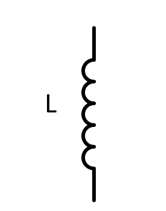Search

# Inductors in EMC - Part 1: Introduction

Updated: Oct 9, 2020

This article explains the basics of inductors, fundamental components in the electromagnetism theory and practice. The fundamental physics laws are reviewed, as well as some examples of inductance in the real world.

## What are inductors?

There are different types of energy: thermal, nuclear, chemical, electric, electromechanical, among others. There are materials, such as magnets, that have inherent magnetic energy. Besides, there are components that can store energy in a magnetic field in a temporary way. These components are called inductors and they exist in a very wide range of shapes and sizes.

## Inductance

A circuit element responding with a counter-voltage to a circulating current, shows inductive properties. This voltage is named self-induction voltage (Uemf) or Electromotive Force (EMF) and it is proportional to the variation speed of the current .L is the inductance in Henry [H], Uemf is the voltage across the inductor and di/dt is the rate of change of current in Amperes per second.

The origin of the letter L comes from Heinrich Lenz, who stated that the induced voltage in a circuit tends to oppose the change in current. The negative sign is due to the opposition. The unit of inductance (L) is Henry [H], [H] = [Vs/A], where [V] stands for voltage, [s] for seconds and [A] for current. The inductance of a coil depends on different factors such as geometry, material, winding turns or the way in which the windings are done.Electromotive Force (UMF) voltage is induced when the switch changes its position.

If the Electromotive Force is caused in the same conductor in which the current varies, we talk about self-inductance. On the other hand, if the force appears in a different conductor, then we talk about mutual inductance . This latter property is of use in transformers, induction cookers or to perform Wireless charging.Comparison between self-inductance and mutual inductance .

## Types of inductors

Inductors are present in the market in a huge variety of sizes, shapes and materials. Even if the physical concept of all these inductors is the same, each type of inductor serves for a different purpose. Specific application of inductors will be reviewed in future articles. The shape and type of an inductor will be given by the magnetic core. All inductors are built placing a single or multiple windings around a core, even if this core is made of air. Typical materials used for cores are ferrite, powdered iron or MnZn. Each material has different permeability (µ), which is the ability of concentrate magnetic flux around the core. Apart from the material, shape is also critical and its selection will depend upon the energy transfer requisites, maximum current, application and electromagnetic emissions, among others. Shape, size, core and winding produce a unique effective permeability.Different types of inductors.

Inductors come in a vast variety of types. In the picture above from left to right: Toroid coil (copper wire winding around a “donut” magnetic core), air core inductor (coil of conducting wire with no magnetic core), PCB surface mount (SMD) power coil inductor with magnetic core, ferrite bead as clamp ferrite (for clamping around a cable), ferrite bead as PCB surface mount (SMD) ferrite bead.

## Real-world inductance

In an ideal world, inductance is purely inductance, with independence of the signal frequency and amplitude circulating through them. In a real world, there are parasitic components due to the physical nature and the way they are built:

• Resistance R. There is a winding of an electrically isolated copper wire with a specific resistance (resistive path)

• Capacitance C. The isolated copper wires are placed close to each other with every winding around a core, building a capacitor (capacitance from wire to wire with the wire isolation as dielectric material)

• Magnetic permeability µr. The core material (magnetic or non-magnetic) changes its magnetic permeability with increasing magnetic flux density and with changing temperatures. This leads to a change in inductance L.

Apart from the inductance value, which limits the maximum energy to be stored, these parasitic components will limit the actual use of an inductor.Ideal inductor.Real inductor.

If we consider the parasitic elements R and C of an inductor, the total impedance Z is as follows :Usually, the parasitic resistance R is quite low, so it can be neglected.At the (parallel) resonant frequency the denominator will be zero, provoking a maximum impedance. That frequency is then:From that point, the capacitive component of the inductance will prevail, and the inductance will not act as an inductance anymore, presenting a capacitive impedance. Therefore, circuits need to be designed to work below the resonance frequency.Impedance Z vs. frequency f of a real inductor with parasitic elements R and C .

## Applications

Inductors are used in many different applications, so only the relevant examples for EMC are listed here:

• Filtering: combinations of inductors, capacitors and resistors are very appropriate to attenuate and remove undesired signals. Inductors play a critical role when pursing Electromagnetic Compatibility (EMC).

• Isolation: the ability to induce currents from one inductor to a different one helps to decouple electrical paths. Transformers are fundamental components in building Switched-Mode Power Supplies (SPMS).

## Conclusion

This article introduced the fundamentals of inductors as well as some of their applications. Inductors are key components in all kind of electronics. In the next two blog posts, we will present some more specific applications of inductors and how they can help you to make your product EMC compliant.

This blog post was contributed by Ignacio de Mendizábal, electronics engineer, Brussels. Many thanks to Ignacio for his valuable work. Reviewed by Reto Keller, electronics engineer, Switzerland.

## References

 Clayton R. Paul. Introduction to electromagnetic compatibility. John Wiley & Sons Inc., 2nd Edition, 2008, p. 337

 Henry W. Ott, Electromagnetic Compatibility Engineering, John Wiley & Sons, Inc., 2009, pp. 52-58